x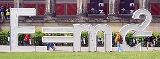Mass-energy equivalenceEncyclopedia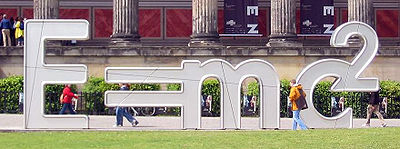In physics
Physics
Physics is a natural science that involves the study of matter and its motion through spacetime, along with related concepts such as energy and force. More broadly, it is the general analysis of nature, conducted in order to understand how the universe behaves.Physics is one of the oldest academic...

, mass–energy equivalence is the concept that the mass
Mass
Mass can be defined as a quantitive measure of the resistance an object has to change in its velocity.In physics, mass commonly refers to any of the following three properties of matter, which have been shown experimentally to be equivalent:...

of a body is a measure of its energy
Energy
In physics, energy is an indirectly observed quantity. It is often understood as the ability a physical system has to do work on other physical systems...

content. In this concept, mass is a property of all energy, and energy is a property of all mass, and the two properties are connected by a constant. This means (for example) that the total internal energy E of a body at rest is equal to the product of its rest mass
Invariant mass
The invariant mass, rest mass, intrinsic mass, proper mass or just mass is a characteristic of the total energy and momentum of an object or a system of objects that is the same in all frames of reference related by Lorentz transformations...

m and a suitable conversion factor
Conversion factor
A conversion factor changes something to a different version or form. A factor is something that brings results or a cause, while conversion is an action of changing the "version" of a thing....

to transform from units of mass to units of energy. Albert Einstein
Albert Einstein
Albert Einstein was a German-born theoretical physicist who developed the theory of general relativity, effecting a revolution in physics. For this achievement, Einstein is often regarded as the father of modern physics and one of the most prolific intellects in human history...

proposed mass–energy equivalence in 1905 in one of his Annus Mirabilis papers
Annus Mirabilis Papers
The Annus Mirabilis papers are the papers of Albert Einstein published in the Annalen der Physik scientific journal in 1905. These four articles contributed substantially to the foundation of modern physics and changed views on space, time, and matter...

entitled "Does the inertia of a body depend upon its energy-content?" The equivalence is described by the famous equation: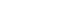where E is energy, m is mass, and c is the speed of light
Speed of light
The speed of light in vacuum, usually denoted by c, is a physical constant important in many areas of physics. Its value is 299,792,458 metres per second, a figure that is exact since the length of the metre is defined from this constant and the international standard for time...

in a vacuum. The formula is dimensionally consistent
Dimensional analysis
In physics and all science, dimensional analysis is a tool to find or check relations among physical quantities by using their dimensions. The dimension of a physical quantity is the combination of the basic physical dimensions which describe it; for example, speed has the dimension length per...

and does not depend on any specific system of measurement units
Systems of measurement
A system of measurement is a set of units which can be used to specify anything which can be measured and were historically important, regulated and defined because of trade and internal commerce...

.
The equation E = mc2 indicates that energy always exhibits relativistic mass in whatever form the energy takes. Mass–energy equivalence does not imply that mass may be "converted" to energy, but it allows for matter to be converted to various types of radiation, and/or various other types of non-material kinetic or potential energy. Through all such conversions, mass remains conserved, since it is a property of matter and any type of energy. In physics, mass must be differentiated from matter. Matter, when seen as certain types of particles, can be created and destroyed (as in particle annihilation
Annihilation
Annihilation is defined as "total destruction" or "complete obliteration" of an object; having its root in the Latin nihil . A literal translation is "to make into nothing"....

or creation
Matter creation
Matter creation is the process inverse to particle annihilation. It is the conversion of massless particles into one or more massive particles. This process is the time reversal of annihilation. Since all known massless particles are bosons and the most familiar massive particles are fermions,...

), but the system of precursors and products of such reactions, as a whole, retain both the original mass and energy, with each of these system properties remaining unchanged (conserved) throughout the process. Letting the m in E = mc2 stand for a quantity of "matter" (rather than mass) may lead to incorrect results, depending on which of several varying definitions of "matter" are chosen.

When energy is removed from a system (for example in binding energy
Binding energy
Binding energy is the mechanical energy required to disassemble a whole into separate parts. A bound system typically has a lower potential energy than its constituent parts; this is what keeps the system together—often this means that energy is released upon the creation of a bound state...

, or the energy given off by an atomic bomb) then mass is always removed along with the energy. This energy retains the missing mass, which will in turn be added to any other system which absorbs it. In this situation E = mc2 can be used to calculate how much mass goes along with the removed energy. It also tells how much mass will be added to any system which later absorbs this energy.

E = mc2 has sometimes been used as an explanation for the origin of energy in nuclear processes, but mass–energy equivalence does not explain the origin of such energies. Instead, this relationship merely indicates that the large amounts of energy released in such reactions may exhibit enough mass that the mass-loss may be measured, when the released energy (and its mass) have been removed from the system. For example, the loss of mass to atoms+neutrons as a result of the capture of a neutron, and loss of a gamma ray, has been used to test mass-energy equivalence to high precision, as the energy of the gamma ray may be compared with the mass defect after capture. In 2005, these were found to agree to 0.0004%, the most precise test of the equivalence of mass and energy to date. This test was performed in the World Year of Physics 2005
World Year of Physics 2005
The year 2005 has been named the World Year of Physics in recognition of the 100th anniversary of Albert Einstein's "Miracle Year," in which he published four landmark papers, and the subsequent advances in the field of physics.-History:...

, a centennial celebration of Einstein's achievements in 1905.

Einstein was not the first to propose a mass–energy relationship (see the History section). However, Einstein was the first scientist to propose the E = mc2 formula and the first to interpret mass–energy equivalence as a fundamental principle that follows from the relativistic symmetries
Spacetime symmetries
Spacetime symmetries are features of spacetime that can be described as exhibiting some form of symmetry. The role of symmetry in physics is important in simplifying solutions to many problems, spacetime symmetries finding ample application in the study of exact solutions of Einstein's field...

of space and time
Spacetime
In physics, spacetime is any mathematical model that combines space and time into a single continuum. Spacetime is usually interpreted with space as being three-dimensional and time playing the role of a fourth dimension that is of a different sort from the spatial dimensions...

.

## Conservation of mass and energy

The concept of mass–energy equivalence connects the concepts of conservation of mass
Conservation of mass
The law of conservation of mass, also known as the principle of mass/matter conservation, states that the mass of an isolated system will remain constant over time...

and conservation of energy
Conservation of energy
The nineteenth century law of conservation of energy is a law of physics. It states that the total amount of energy in an isolated system remains constant over time. The total energy is said to be conserved over time...

, which continue to hold separately in any isolated system
Isolated system
In the natural sciences an isolated system, as contrasted with an open system, is a physical system without any external exchange. If it has any surroundings, it does not interact with them. It obeys in particular the first of the conservation laws: its total energy - mass stays constant...

(one that is closed to loss of any type of energy, including energy associated with loss of matter). The theory of relativity allows particles which have rest mass to be converted to other forms of mass which require motion, such as kinetic energy
Kinetic energy
The kinetic energy of an object is the energy which it possesses due to its motion.It is defined as the work needed to accelerate a body of a given mass from rest to its stated velocity. Having gained this energy during its acceleration, the body maintains this kinetic energy unless its speed changes...

, heat, or light. However, the system mass remains. Kinetic energy or light can also be converted to new kinds of particles which have rest mass
Mass
Mass can be defined as a quantitive measure of the resistance an object has to change in its velocity.In physics, mass commonly refers to any of the following three properties of matter, which have been shown experimentally to be equivalent:...

, but again the energy remains. Both the total mass and the total energy inside an isolated system remain constant over time, as seen by any single observer in a given inertial frame.

In other words, energy can neither be created nor destroyed, and energy, in all of its forms, has mass. Mass also can neither be created nor destroyed, and in all of its forms, has energy. According to the theory of relativity, mass and energy as commonly understood, are two names for the same thing, and neither one is changed nor transformed into the other. Rather, neither one exists without the other existing also, as a property of a system. Rather than mass being changed into energy, the view of special relativity
Special relativity
Special relativity is the physical theory of measurement in an inertial frame of reference proposed in 1905 by Albert Einstein in the paper "On the Electrodynamics of Moving Bodies".It generalizes Galileo's...

is that rest mass has been changed to a more mobile form of mass, but remains mass. In the transformation process, neither the amount of mass nor the amount of energy changes, since both are properties which are connected to each other via a simple constant. Thus, if energy leaves a system by changing its form, it simply takes its system mass with it. This view requires that if either mass or energy disappears from a system, it will always be found that both have simply moved off to another place, where they may both be measured as an increase of both mass and energy corresponding to the loss in the first system.

### Fast-moving objects and systems of objects

When an object is pulled in the direction of motion, it gains momentum and energy, but when the object is already traveling near the speed of light
Speed of light
The speed of light in vacuum, usually denoted by c, is a physical constant important in many areas of physics. Its value is 299,792,458 metres per second, a figure that is exact since the length of the metre is defined from this constant and the international standard for time...

, it cannot move much faster, no matter how much energy it absorbs. Its momentum and energy continue to increase without bounds, whereas its speed approaches a constant value—the speed of light. This implies that in relativity the momentum
Momentum
In classical mechanics, linear momentum or translational momentum is the product of the mass and velocity of an object...

of an object cannot be a constant times the velocity
Velocity
In physics, velocity is speed in a given direction. Speed describes only how fast an object is moving, whereas velocity gives both the speed and direction of the object's motion. To have a constant velocity, an object must have a constant speed and motion in a constant direction. Constant ...

, nor can the kinetic energy be a constant times the square of the velocity.

A property called the relativistic mass is defined as the ratio of the momentum of an object to its velocity. Relativistic mass depends on the motion of the object, so that different observers in relative motion see different values for it. If the object is moving slowly, the relativistic mass is nearly equal to the rest mass and both are nearly equal to the usual Newtonian mass. If the object is moving quickly, the relativistic mass is greater than the rest mass by an amount equal to the mass associated with the kinetic energy
Kinetic energy
The kinetic energy of an object is the energy which it possesses due to its motion.It is defined as the work needed to accelerate a body of a given mass from rest to its stated velocity. Having gained this energy during its acceleration, the body maintains this kinetic energy unless its speed changes...

of the object. As the object approaches the speed of light, the relativistic mass grows infinitely, because the kinetic energy
Kinetic energy
The kinetic energy of an object is the energy which it possesses due to its motion.It is defined as the work needed to accelerate a body of a given mass from rest to its stated velocity. Having gained this energy during its acceleration, the body maintains this kinetic energy unless its speed changes...

grows infinitely and this energy is associated with mass.

The relativistic mass is always equal to the total energy (rest energy plus kinetic energy) divided by c2. Because the relativistic mass is exactly proportional to the energy, relativistic mass and relativistic energy are nearly synonyms; the only difference between them is the units. If length and time are measured in natural units
Natural units
In physics, natural units are physical units of measurement based only on universal physical constants. For example the elementary charge e is a natural unit of electric charge, or the speed of light c is a natural unit of speed...

, the speed of light is equal to 1, and even this difference disappears. Then mass and energy have the same units and are always equal, so it is redundant to speak about relativistic mass, because it is just another name for the energy. This is why physicists usually reserve the useful short word "mass" to mean rest-mass, or invariant mass
Invariant mass
The invariant mass, rest mass, intrinsic mass, proper mass or just mass is a characteristic of the total energy and momentum of an object or a system of objects that is the same in all frames of reference related by Lorentz transformations...

, and not relativistic mass.

The relativistic mass of a moving object is larger than the relativistic mass of an object that is not moving, because a moving object has extra kinetic energy. The rest mass of an object is defined as the mass of an object when it is at rest, so that the rest mass is always the same, independent of the motion of the observer: it is the same in all inertial frames.

For things and systems made up of many parts, like an atomic nucleus
Atomic nucleus
The nucleus is the very dense region consisting of protons and neutrons at the center of an atom. It was discovered in 1911, as a result of Ernest Rutherford's interpretation of the famous 1909 Rutherford experiment performed by Hans Geiger and Ernest Marsden, under the direction of Rutherford. The...

, planet
Planet
A planet is a celestial body orbiting a star or stellar remnant that is massive enough to be rounded by its own gravity, is not massive enough to cause thermonuclear fusion, and has cleared its neighbouring region of planetesimals.The term planet is ancient, with ties to history, science,...

, or star
Star
A star is a massive, luminous sphere of plasma held together by gravity. At the end of its lifetime, a star can also contain a proportion of degenerate matter. The nearest star to Earth is the Sun, which is the source of most of the energy on Earth...

, the relativistic mass is the sum of the relativistic masses (or energies) of the parts, because energies are additive in closed systems. This is not true in systems which are open, however, if energy is subtracted. For example, if a system is bound by attractive forces, and the work the forces do in attraction is removed from the system, then mass will be lost with this removed energy. Such work is a form of energy which itself has mass, and thus mass is removed from the system, as it is bound. For example, the mass of an atomic nucleus is less than the total mass of the protons and neutrons that make it up, but this is only true after the energy (work) of binding has been removed in the form of a gamma ray (which in this system, carries away the mass of binding). This mass decrease is also equivalent to the energy required to break up the nucleus into individual protons and neutrons (in this case, work and mass would need to be supplied). Similarly, the mass of the solar system is slightly less than the masses of sun and planets individually.

For a system of particles going off in different directions, the invariant mass
Invariant mass
The invariant mass, rest mass, intrinsic mass, proper mass or just mass is a characteristic of the total energy and momentum of an object or a system of objects that is the same in all frames of reference related by Lorentz transformations...

of the system is the analog of the rest mass, and is the same for all observers, even those in relative motion. It is defined as the total energy (divided by c2) in the center of mass frame (where by definition, the system total momentum is zero). A simple example of an object with moving parts but zero total momentum, is a container of gas. In this case, the mass of the container is given by its total energy (including the kinetic energy of the gas molecules), since the system total energy and invariant mass are the same in any reference frame where the momentum is zero, and such a reference frame is also the only frame in which the object can be weighed. In a similar way, the theory of special relativity posits that the thermal energy in all objects (including solids) contributes to their total masses and weights, even though this energy is present as the kinetic and potential energies of the atoms in the object, and is (in a similar way to the gas) is not seen in the rest masses of the atoms that make up the object.

In a similar manner, even photons (light quanta), if trapped in a container space (as a photon gas
Photon gas
In physics, a photon gas is a gas-like collection of photons, which has many of the same properties of a conventional gas like hydrogen or neon - including pressure, temperature, and entropy...

Thermal radiation is electromagnetic radiation generated by the thermal motion of charged particles in matter. All matter with a temperature greater than absolute zero emits thermal radiation....

), would contribute a mass associated with their energy to the container. Such an extra mass, in theory, could be weighed in the same way as any other type of rest mass. This is true in special relativity theory, even though individually, photons have no rest mass. The property that trapped energy in any form adds weighable mass to systems that have no net momentum, is one of the characteristic and notable consequences of relativity. It has no classical counterpart in classical Newtonian physics, in which radiation, light, heat, and kinetic energy never exhibit weighable mass under any circumstances.

## Applicability of the strict mass–energy equivalence formula, E = mc²

As is noted above, two different definitions of mass have been used in special relativity, and also two different definitions of energy. The simple equation E = mc² is not generally applicable to all these types of mass and energy, except in the special case that the momentum is zero for the system under consideration. In such a case, which is always guaranteed when observing the system from the center of mass frame, E = mc² is true for any type of mass and energy that are chosen. Thus, for example, in the center of mass frame the total energy of an object or system is equal to its rest mass times c², a useful equality. This is the relationship used for the container of gas in the previous example. It is not true in other reference frames in which a system or object's total energy will depend on both its rest (or invariant) mass, and also its total momentum.

In inertial reference frames other than the rest frame or center of mass frame, the equation E = mc² remains true if the energy is the relativistic energy and the mass the relativistic mass. It is also correct if the energy is the rest or invariant energy (also the minimum energy), and the mass is the rest or invariant mass.

However, connection of the total or relativistic energy (Er) with the rest or invariant mass (m0) requires consideration of the system total momentum, in systems and reference frames where momentum has a non-zero value. The formula then required to connect the different kinds of mass and energy, is the extended version of Einstein's equation, called the relativistic energy–momentum relationship: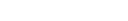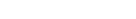or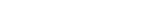Here the (pc)2 term represents the square of the Euclidean norm (total vector length) of the various momentum vectors in the system, which reduces to the square of the simple momentum magnitude, if only a single particle is considered. This equation reduces to E = mc² when the momentum term is zero. For photons where m0 = 0, the equation reduces to Er = pc.

## Meanings of the strict mass–energy equivalence formula, E = mc²Mass–energy equivalence states that any object has a certain energy, even when it is stationary. In Newtonian mechanics, a motionless body has no kinetic energy
Kinetic energy
The kinetic energy of an object is the energy which it possesses due to its motion.It is defined as the work needed to accelerate a body of a given mass from rest to its stated velocity. Having gained this energy during its acceleration, the body maintains this kinetic energy unless its speed changes...

, and it may or may not have other amounts of internal stored energy, like chemical energy
Chemical energy
Chemical energy is the potential of a chemical substance to undergo a transformation through a chemical reaction or, to transform other chemical substances...

or thermal energy
Thermal energy
Thermal energy is the part of the total internal energy of a thermodynamic system or sample of matter that results in the system's temperature....

, in addition to any potential energy
Potential energy
In physics, potential energy is the energy stored in a body or in a system due to its position in a force field or due to its configuration. The SI unit of measure for energy and work is the Joule...

it may have from its position in a field of force
Field (physics)
In physics, a field is a physical quantity associated with each point of spacetime. A field can be classified as a scalar field, a vector field, a spinor field, or a tensor field according to whether the value of the field at each point is a scalar, a vector, a spinor or, more generally, a tensor,...

. In Newtonian mechanics, all of these energies are much smaller than the mass of the object times the speed of light squared.

In relativity, all of the energy that moves along with an object (that is, all the energy which is present in the object's rest frame) contributes to the total mass of the body, which measures how much it resists acceleration. Each potential and kinetic energy makes a proportional contribution to the mass. As noted above, even if a box of ideal mirrors "contains" light, then the individually massless photons still contribute to the total mass of the box, by the amount of their energy divided by c2.

In relativity
Theory of relativity
The theory of relativity, or simply relativity, encompasses two theories of Albert Einstein: special relativity and general relativity. However, the word relativity is sometimes used in reference to Galilean invariance....

, removing energy is removing mass, and for an observer in the center of mass frame, the formula m = E/c² indicates how much mass is lost when energy is removed. In a chemical or nuclear reaction, the mass of the atoms that come out is less than the mass of the atoms that go in, and the difference in mass shows up as heat and light which has the same relativistic mass as the difference (and also the same invariant mass
Invariant mass
The invariant mass, rest mass, intrinsic mass, proper mass or just mass is a characteristic of the total energy and momentum of an object or a system of objects that is the same in all frames of reference related by Lorentz transformations...

in the center of mass frame of the system). In this case, the E in the formula is the energy released and removed, and the mass m is how much the mass decreases. In the same way, when any sort of energy is added to an isolated system, the increase in the mass is equal to the added energy divided by c². For example, when water is heated it gains about of mass for every joule of heat added to the water.

An object moves with different speed in different frames, depending on the motion of the observer, so the kinetic energy in both Newtonian mechanics and relativity is frame dependent. This means that the amount of relativistic energy, and therefore the amount of relativistic mass, that an object is measured to have depends on the observer. The rest mass is defined as the mass that an object has when it is not moving (or when an inertial frame is chosen such that it is not moving). The term also applies to the invariant mass of systems when the system as a whole is not "moving" (has no net momentum). The rest and invariant masses are the smallest possible value of the mass of the object or system. They also are conserved quantities, so long as the system is closed. Because of the way they are calculated, the effects of moving observers are subtracted, so these quantities do not change with the motion of the observer.

The rest mass is almost never additive: the rest mass of an object is not the sum of the rest masses of its parts. The rest mass of an object is the total energy of all the parts, including kinetic energy, as measured by an observer that sees the center of the mass of the object to be standing still. The rest mass adds up only if the parts are standing still and do not attract or repel, so that they do not have any extra kinetic or potential energy. The other possibility is that they have a positive kinetic energy and a negative potential energy that exactly cancels.

### Binding energy and the "mass defect"

Whenever any type of energy is removed from a system, the mass associated with the energy is also removed, and the system therefore loses mass. This mass defect in the system may be simply calculated as Δm = ΔE/c2, but use of this formula in such circumstances has led to the false idea that mass has been "converted" to energy. This may be particularly the case when the energy (and mass) removed from the system is associated with the binding energy of the system. In such cases, the binding energy is observed as a "mass defect" or deficit in the new system and the fact that the released energy is not easily weighed may cause its mass to be neglected.

The difference between the rest mass of a bound system and of the unbound parts is the binding energy
Binding energy
Binding energy is the mechanical energy required to disassemble a whole into separate parts. A bound system typically has a lower potential energy than its constituent parts; this is what keeps the system together—often this means that energy is released upon the creation of a bound state...

of the system, if this energy has been removed after binding. For example, a water molecule weighs a little less than two free hydrogen atoms and an oxygen atom; the minuscule mass difference is the energy that is needed to split the molecule into three individual atoms (divided by c²), and which was given off as heat when the molecule formed (this heat had mass). Likewise, a stick of dynamite in theory weighs a little bit more than the fragments after the explosion, but this is true only so long as the fragments are cooled and the heat removed. In this case the mass difference is the energy/heat that is released when the dynamite explodes, and when this heat escapes, the mass associated with it escapes, only to be deposited in the surroundings which absorb the heat (so that total mass is conserved).

Such a change in mass may only happen when the system is open, and the energy and mass escapes. Thus, if a stick of dynamite is blown up in a hermetically sealed chamber, the mass of the chamber and fragments, the heat, sound, and light would still be equal to the original mass of the chamber and dynamite. If sitting on a scale, the weight and mass would not change. This would in theory also happen even with a nuclear bomb, if it could be kept in an ideal box of infinite strength, which did not rupture or pass radiation. Thus, a 21.5 kiloton
TNT equivalent
TNT equivalent is a method of quantifying the energy released in explosions. The ton of TNT is a unit of energy equal to 4.184 gigajoules, which is approximately the amount of energy released in the detonation of one ton of TNT...

(9 x 1013joule) nuclear bomb produces about one gram of heat and electromagnetic radiation, but the mass of this energy would not be detectable in an exploded bomb in an ideal box sitting on a scale; instead, the contents of the box would be heated to millions of degrees without changing total mass and weight. If then, however, a transparent window (passing only electromagnetic radiation) were opened in such an ideal box after the explosion, and a beam of X-rays and other lower-energy light allowed to escape the box, it would eventually be found to weigh one gram less than it had before the explosion. This weight-loss and mass-loss would happen as the box was cooled by this process, to room temperature. However, any surrounding mass which had absorbed the X-rays (and other "heat") would gain this gram of mass from the resulting heating, so the mass "loss" would represent merely its relocation. Thus, no mass (or, in the case of a nuclear bomb, no matter) would be "converted" to energy in such a process. Mass and energy, as always, would both be separately conserved.

### Massless particles

Massless particles have zero rest mass. Their relativistic mass is simply their relativistic energy, divided by c2, or m(relativistic) = E/c2. The energy for photons is E = hf where h is Planck's constant and f is the photon frequency. This frequency and thus the relativistic energy are frame-dependent.

If an observer runs away from a photon in the direction it travels from a source, having it catch up with the observer, then when the photon catches up it will be seen as having less energy than it had at the source. The faster the observer is traveling with regard to the source when the photon catches up, the less energy the photon will have. As an observer approaches the speed of light with regard to the source, the photon looks redder and redder, by relativistic Doppler effect
Relativistic Doppler effect
The relativistic Doppler effect is the change in frequency of light, caused by the relative motion of the source and the observer , when taking into account effects described by the special theory of relativity.The relativistic Doppler effect is different from the non-relativistic Doppler effect...

(the Doppler shift is the relativistic formula), and the energy of a very long-wavelength photon approaches zero. This is why a photon is massless; this means that the rest mass of a photon is zero.

Two photons moving in different directions cannot both be made to have arbitrarily small total energy by changing frames, or by moving toward or away from them. The reason is that in a two-photon system, the energy of one photon is decreased by chasing after it, but the energy of the other will increase with the same shift in observer motion. Two photons not moving in the same direction will exhibit an inertial frame where the combined energy is smallest, but not zero. This is called the center of mass
Center of mass
In physics, the center of mass or barycenter of a system is the average location of all of its mass. In the case of a rigid body, the position of the center of mass is fixed in relation to the body...

frame or the center of momentum frame; these terms are almost synonyms (the center of mass frame is the special case of a center of momentum frame where the center of mass is put at the origin). The most that chasing a pair of photons can accomplish to decrease their energy is to put the observer in frame where the photons have equal energy and are moving directly away from each other. In this frame, the observer is now moving in the same direction and speed as the center of mass of the two photons. The total momentum of the photons is now zero, since their momentums are equal and opposite. In this frame the two photons, as a system, have a mass equal to their total energy divided by c2. This mass is called the invariant mass
Invariant mass
The invariant mass, rest mass, intrinsic mass, proper mass or just mass is a characteristic of the total energy and momentum of an object or a system of objects that is the same in all frames of reference related by Lorentz transformations...

of the pair of photons together. It is the smallest mass and energy the system may be seen to have, by any observer. It is only the invariant mass of a two-photon system that can be used to make a single particle with the same rest mass.

If the photons are formed by the collision of a particle and an antiparticle, the invariant mass is the same as the total energy of the particle and antiparticle (their rest energy plus the kinetic energy), in the center of mass frame, where they will automatically be moving in equal and opposite directions (since they have equal momentum in this frame). If the photons are formed by the disintegration of a single particle with a well-defined rest mass, like the neutral pion
Pion
In particle physics, a pion is any of three subatomic particles: , , and . Pions are the lightest mesons and they play an important role in explaining the low-energy properties of the strong nuclear force....

, the invariant mass of the photons is equal to rest mass of the pion. In this case, the center of mass frame for the pion is just the frame where the pion is at rest, and the center of mass does not change after it disintegrates into two photons. After the two photons are formed, their center of mass is still moving the same way the pion did, and their total energy in this frame adds up to the mass energy of the pion. Thus, by calculating the invariant mass
Invariant mass
The invariant mass, rest mass, intrinsic mass, proper mass or just mass is a characteristic of the total energy and momentum of an object or a system of objects that is the same in all frames of reference related by Lorentz transformations...

of pairs of photons in a particle detector, pairs can be identified that were probably produced by pion disintegration.

## Consequences for nuclear physicsMax Planck
Max Planck
Max Karl Ernst Ludwig Planck, ForMemRS, was a German physicist who actualized the quantum physics, initiating a revolution in natural science and philosophy. He is regarded as the founder of the quantum theory, for which he received the Nobel Prize in Physics in 1918.-Life and career:Planck came...

pointed out that the mass–energy equivalence formula implied that bound systems would have a mass less than the sum of their constituents, once the binding energy had been allowed to escape. However, Planck was thinking about chemical reactions, where the binding energy is too small to measure. Einstein suggested that radioactive materials such as radium
Radium is a chemical element with atomic number 88, represented by the symbol Ra. Radium is an almost pure-white alkaline earth metal, but it readily oxidizes on exposure to air, becoming black in color. All isotopes of radium are highly radioactive, with the most stable isotope being radium-226,...

would provide a test of the theory, but even though a large amount of energy is released per atom in radium, due to the half-life
Half-life
Half-life, abbreviated t½, is the period of time it takes for the amount of a substance undergoing decay to decrease by half. The name was originally used to describe a characteristic of unstable atoms , but it may apply to any quantity which follows a set-rate decay.The original term, dating to...

of the substance (1602 years), only a small fraction of radium atoms decay over experimentally measurable period of time.

Once the nucleus was discovered, experimenters realized that the very high binding energies of the atomic nuclei should allow calculation of their binding energies, simply from mass differences. But it was not until the discovery of the neutron
Neutron
The neutron is a subatomic hadron particle which has the symbol or , no net electric charge and a mass slightly larger than that of a proton. With the exception of hydrogen, nuclei of atoms consist of protons and neutrons, which are therefore collectively referred to as nucleons. The number of...

in 1932, and the measurement of the neutron mass, that this calculation could actually be performed (see nuclear binding energy
Nuclear binding energy
Nuclear binding energy is the energy required to split a nucleus of an atom into its component parts. The component parts are neutrons and protons, which are collectively called nucleons...

for example calculation). A little while later, the first transmutation
Nuclear transmutation
Nuclear transmutation is the conversion of one chemical element or isotope into another. In other words, atoms of one element can be changed into atoms of other element by 'transmutation'...

reactions (such as the Cockcroft-Walton experiment: 7Li + p → 2 4He) verified Einstein's formula to an accuracy of ±0.5%.
In 2005, Rainville et al. published a direct test of the energy-equivalence of mass lost in the binding-energy of a neutron to atoms of particular isotopes of silicon and sulfur, by comparing the mass-lost to the energy of the emitted gamma ray associated with the neutron capture. The binding mass-loss agreed with the gamma ray energy to a precision of ±0.00004 %, the most accurate test of E=mc2 to date.

The mass–energy equivalence formula was used in the development of the atomic bomb. By measuring the mass of different atomic nuclei and subtracting from that number the total mass of the protons and neutrons as they would weigh separately, one gets the exact binding energy
Binding energy
Binding energy is the mechanical energy required to disassemble a whole into separate parts. A bound system typically has a lower potential energy than its constituent parts; this is what keeps the system together—often this means that energy is released upon the creation of a bound state...

available in an atomic nucleus
Atomic nucleus
The nucleus is the very dense region consisting of protons and neutrons at the center of an atom. It was discovered in 1911, as a result of Ernest Rutherford's interpretation of the famous 1909 Rutherford experiment performed by Hans Geiger and Ernest Marsden, under the direction of Rutherford. The...

. This is used to calculate the energy released in any nuclear reaction
Nuclear reaction
In nuclear physics and nuclear chemistry, a nuclear reaction is semantically considered to be the process in which two nuclei, or else a nucleus of an atom and a subatomic particle from outside the atom, collide to produce products different from the initial particles...

, as the difference in the total mass of the nuclei that enter and exit the reaction.

## Practical examples

Einstein used the CGS system of units (centimeters, grams, seconds, dynes, and ergs), but the formula is independent of the system of units. In natural units
Natural units
In physics, natural units are physical units of measurement based only on universal physical constants. For example the elementary charge e is a natural unit of electric charge, or the speed of light c is a natural unit of speed...

, the speed of light is defined to equal 1, and the formula expresses an identity: E = m. In the SI
International System of Units
The International System of Units is the modern form of the metric system and is generally a system of units of measurement devised around seven base units and the convenience of the number ten. The older metric system included several groups of units...

system (expressing the ratio E / m in joules per kilogram using the value of c in meters per second
Metre per second
Metre per second is an SI derived unit of both speed and velocity , defined by distance in metres divided by time in seconds....

):
E / m = c2 = (299,792,458 m/s)2 = 89,875,517,873,681,764 J/kg (≈9.0 × 1016 joules per kilogram)

So the energy equivalent of one gram
Gram
The gram is a metric system unit of mass....

of mass is equivalent to:
89.9 terajoules
25.0 million kilowatt-hours (≈25 GW·h)
21.5 billion kilocalories
Calorie
The calorie is a pre-SI metric unit of energy. It was first defined by Nicolas Clément in 1824 as a unit of heat, entering French and English dictionaries between 1841 and 1867. In most fields its use is archaic, having been replaced by the SI unit of energy, the joule...

(≈21 Tcal)
85.2 billion BTUs
British thermal unit
The British thermal unit is a traditional unit of energy equal to about 1055 joules. It is approximately the amount of energy needed to heat of water, which is exactly one tenth of a UK gallon or about 0.1198 US gallons, from 39°F to 40°F...

or to the energy released by combustion of the following:
21.5 kilotons of TNT-equivalent
TNT equivalent
TNT equivalent is a method of quantifying the energy released in explosions. The ton of TNT is a unit of energy equal to 4.184 gigajoules, which is approximately the amount of energy released in the detonation of one ton of TNT...

energy (≈21 kt)
568,000 US gallons of automotive gasoline

Any time energy is generated, the process can be evaluated from an E = mc2 perspective. For instance, the "Gadget
Fat Man
"Fat Man" is the codename for the atomic bomb that was detonated over Nagasaki, Japan, by the United States on August 9, 1945. It was the second of the only two nuclear weapons to be used in warfare to date , and its detonation caused the third man-made nuclear explosion. The name also refers more...

"-style bomb used in the Trinity test
Trinity test
Trinity was the code name of the first test of a nuclear weapon. This test was conducted by the United States Army on July 16, 1945, in the Jornada del Muerto desert about 35 miles southeast of Socorro, New Mexico, at the new White Sands Proving Ground, which incorporated the Alamogordo Bombing...

and the bombing of Nagasaki had an explosive yield equivalent to 21 kt of TNT. About 1 kg of the approximately 6.15 kg of plutonium in each of these bombs fissioned into lighter elements totaling almost exactly one gram less, after cooling [The electromagnetic radiation and kinetic energy (thermal and blast energy) released in this explosion carried the missing one gram of mass.] This occurs because nuclear binding energy
Binding energy
Binding energy is the mechanical energy required to disassemble a whole into separate parts. A bound system typically has a lower potential energy than its constituent parts; this is what keeps the system together—often this means that energy is released upon the creation of a bound state...

is released whenever elements with more than 62 nucleons fission.

Another example is hydroelectric generation
Hydroelectricity
Hydroelectricity is the term referring to electricity generated by hydropower; the production of electrical power through the use of the gravitational force of falling or flowing water. It is the most widely used form of renewable energy...

. The electrical energy produced by Grand Coulee Dam's
Grand Coulee Dam
Grand Coulee Dam is a gravity dam on the Columbia River in the U.S. state of Washington built to produce hydroelectric power and provide irrigation. It was constructed between 1933 and 1942, originally with two power plants. A third power station was completed in 1974 to increase its energy...

turbines
Water turbine
A water turbine is a rotary engine that takes energy from moving water.Water turbines were developed in the 19th century and were widely used for industrial power prior to electrical grids. Now they are mostly used for electric power generation. They harness a clean and renewable energy...

every 3.7 hours represents one gram of mass. This mass passes to the electrical devices which are powered by the generators (such as lights in cities), where it appears as a gram of heat and light. Turbine designers look at their equations in terms of pressure, torque, and RPM. However, Einstein's equations show that all energy has mass, and thus the electrical energy produced by a dam's generators, and the heat and light which result from it, all retain their mass, which is equivalent to the energy. The potential energy—and equivalent mass—represented by the waters of the Columbia River
Columbia River
The Columbia River is the largest river in the Pacific Northwest region of North America. The river rises in the Rocky Mountains of British Columbia, Canada, flows northwest and then south into the U.S. state of Washington, then turns west to form most of the border between Washington and the state...

as it descends to the Pacific Ocean would be converted to heat due to viscous friction and the turbulence
Turbulence
In fluid dynamics, turbulence or turbulent flow is a flow regime characterized by chaotic and stochastic property changes. This includes low momentum diffusion, high momentum convection, and rapid variation of pressure and velocity in space and time...

of white water rapids and waterfalls were it not for the dam and its generators. This heat would remain as mass on site at the water, were it not for the equipment which converted some of this potential and kinetic energy into electrical energy, which can be moved from place to place (taking mass with it).

Whenever energy is added to a system, the system gains mass.
• A spring's mass increases whenever it is put into compression or tension. Its added mass arises from the added potential energy stored within it, which is bound in the stretched chemical (electron) bonds linking the atoms within the spring.
• Raising the temperature of an object (increasing its heat energy) increases its mass. For example, consider the world's primary mass standard for the kilogram, made of platinum/iridium. If its temperature is allowed to change by 1°C, its mass will change by 1.5 picograms (1 pg = 1 × 10−12 g).
• A spinning ball will weigh more than a ball that is not spinning. Its increase of mass is exactly the equivalent of the mass of energy of rotation, which is itself the sum of the kinetic energies of all the moving parts of the ball. For example, the Earth itself is more massive due to its daily rotation, than it would be with no rotation. This rotational energy (2.14 x 1029 J) represents 2.38 billion metric tons of added mass.

Note that no net mass or energy is really created or lost in any of these examples and scenarios. Mass/energy simply moves from one place to another. These are some examples of the transfer of energy and mass in accordance with the principle of mass–energy conservation.

Note further that in accordance with Einstein's Strong Equivalence Principle (SEP), all forms of mass and energy produce a gravitational field in the same way. So all radiated and transmitted energy retains its mass. Not only does the matter comprising Earth create gravity, but the gravitational field itself has mass, and that mass contributes to the field too. This effect is accounted for in ultra-precise laser ranging to the Moon as the Earth orbits the Sun when testing Einstein's general theory of relativity.

According to E=mc2, no closed system (any system treated and observed as a whole) ever loses mass, even when rest mass is converted to energy. All types of energy contribute to mass, including potential energies. In relativity, interaction potentials are always due to local fields
Field (physics)
In physics, a field is a physical quantity associated with each point of spacetime. A field can be classified as a scalar field, a vector field, a spinor field, or a tensor field according to whether the value of the field at each point is a scalar, a vector, a spinor or, more generally, a tensor,...

, not to direct nonlocal interactions, because signals cannot travel faster than light. The field energy is stored in field gradients or, in some cases (for massive fields), where the field has a nonzero value. The mass associated with the potential energy is the mass–energy of the field energy. The mass associated with field energy can be detected, in principle, by gravitational experiments, by checking how the field attracts other objects gravitationally.

The energy in the gravitational field itself has some differences from other energies. There are several consistent ways to define the location of the energy in a gravitational field, all of which agree on the total energy when space is mostly flat and empty. But because the gravitational field can be made to vanish locally at any point by choosing a free-falling frame, the precise location of the energy becomes dependent on the observer's frame of reference, and thus has no exact location, even though it exists somewhere for any given observer. In the limit for low field strengths, this gravitational field energy is the familiar Newtonian gravitational potential energy.

## Efficiency

Although mass cannot be converted to energy, matter particles can be. Also, a certain amount of the ill-defined "matter" in ordinary objects can be converted to active energy (light and heat), even though no identifiable real particles are destroyed. Such conversions happen in nuclear weapons, in which the protons and neutrons in atomic nuclei lose a small fraction of their average mass, but this mass-loss is not due to the destruction of any protons or neutrons (or even, in general, lighter particles like electrons). Also the mass is not destroyed, but simply removed from the system in the form of heat and light from the reaction.

In nuclear reactions, typically only a small fraction of the total mass–energy of the bomb is converted into heat, light, radiation and motion, which are "active" forms which can be used. When an atom fissions, it loses only about 0.1% of its mass (which escapes from the system and does not disappear), and in a bomb or reactor not all the atoms can fission. In a fission based atomic bomb, the efficiency is only 40%, so only 40% of the fissionable atoms actually fission, and only 0.04% of the total mass appears as energy in the end. In nuclear fusion, more of the mass is released as usable energy, roughly 0.3%. But in a fusion bomb (see nuclear weapon yield
Nuclear weapon yield
The explosive yield of a nuclear weapon is the amount of energy discharged when a nuclear weapon is detonated, expressed usually in the equivalent mass of trinitrotoluene , either in kilotons or megatons , but sometimes also in terajoules...

), the bomb mass is partly casing and non-reacting components, so that in practicality, no more than about 0.03% of the total mass of the entire weapon is released as usable energy (which, again, retains the "missing" mass).

In theory, it should be possible to convert all of the mass in matter into heat and light (which would of course have the same mass), but none of the theoretically known methods are practical. One way to convert all matter into usable energy is to annihilate matter with antimatter
Antimatter
In particle physics, antimatter is the extension of the concept of the antiparticle to matter, where antimatter is composed of antiparticles in the same way that normal matter is composed of particles...

. But antimatter is rare in our universe
Baryon asymmetry
The baryon asymmetry problem in physics refers to the apparent fact that there is an imbalance in baryonic matter and antibaryonic matter in the universe. Neither the standard model of particle physics, nor the theory of general relativity provide an obvious explanation for why this should be so;...

, and must be made first. Due to inefficient mechanisms of production, making antimatter always requires far more energy than would be released when it was annihilated.

Since most of the mass of ordinary objects resides in protons and neutrons, in order to convert all ordinary matter to useful energy, the protons and neutrons must be converted to lighter particles. In the standard model of particle physics
Standard Model
The Standard Model of particle physics is a theory concerning the electromagnetic, weak, and strong nuclear interactions, which mediate the dynamics of the known subatomic particles. Developed throughout the mid to late 20th century, the current formulation was finalized in the mid 1970s upon...

, the number of protons plus neutrons is nearly exactly conserved. Still, Gerard 't Hooft showed that there is a process which will convert protons and neutrons to antielectrons and neutrinos. This is the weak SU(2) instanton
Instanton
An instanton is a notion appearing in theoretical and mathematical physics. Mathematically, a Yang–Mills instanton is a self-dual or anti-self-dual connection in a principal bundle over a four-dimensional Riemannian manifold that plays the role of physical space-time in non-abelian gauge theory...

proposed by Belavin Polyakov Schwarz and Tyupkin. This process, can in principle convert all the mass of matter into neutrinos and usable energy, but it is normally extraordinarily slow. Later it became clear that this process will happen at a fast rate at very high temperatures, since then instanton-like configurations will be copiously produced from thermal fluctuations
Statistical mechanics
Statistical mechanics or statistical thermodynamicsThe terms statistical mechanics and statistical thermodynamics are used interchangeably...

. The temperature required is so high that it would only have been reached shortly after the big bang
Big Bang
The Big Bang theory is the prevailing cosmological model that explains the early development of the Universe. According to the Big Bang theory, the Universe was once in an extremely hot and dense state which expanded rapidly. This rapid expansion caused the young Universe to cool and resulted in...

.

Many extensions of the standard model contain magnetic monopole
Magnetic monopole
A magnetic monopole is a hypothetical particle in particle physics that is a magnet with only one magnetic pole . In more technical terms, a magnetic monopole would have a net "magnetic charge". Modern interest in the concept stems from particle theories, notably the grand unified and superstring...

s, and in some models of grand unification
Grand unification theory
The term Grand Unified Theory, often abbreviated as GUT, refers to any of several similar candidate models in particle physics in which at high-energy, the three gauge interactions of the Standard Model which define the electromagnetic, weak, and strong interactions, are merged into one single...

, these monopoles catalyze proton decay
Proton decay
In particle physics, proton decay is a hypothetical form of radioactive decay in which the proton decays into lighter subatomic particles, such as a neutral pion and a positron...

, a process known as the Callan–Rubakov effect. This process would be an efficient mass–energy conversion at ordinary temperatures, but it requires making monopole
Magnetic monopole
A magnetic monopole is a hypothetical particle in particle physics that is a magnet with only one magnetic pole . In more technical terms, a magnetic monopole would have a net "magnetic charge". Modern interest in the concept stems from particle theories, notably the grand unified and superstring...

s and anti-monopoles first. The energy required to produce monopoles is believed to be enormous, but magnetic charge is conserved, so that the lightest monopole is stable. All these properties are deduced in theoretical models—magnetic monopoles have never been observed, nor have they been produced in any experiment so far.

A third known method of total matter–energy conversion is using gravity, specifically black holes. Stephen Hawking
Stephen Hawking
Stephen William Hawking, CH, CBE, FRS, FRSA is an English theoretical physicist and cosmologist, whose scientific books and public appearances have made him an academic celebrity...

theorized that black holes radiate thermally with no regard to how they are formed. So it is theoretically possible to throw matter into a black hole and use the emitted heat to generate power. According to the theory of Hawking radiation
Hawking radiation is a thermal radiation with a black body spectrum predicted to be emitted by black holes due to quantum effects. It is named after the physicist Stephen Hawking, who provided a theoretical argument for its existence in 1974, and sometimes also after the physicist Jacob Bekenstein...

, however, the black hole used will radiate at a higher rate the smaller it is, producing usable powers at only small black hole masses, where usable may for example be something greater than the local background radiation. It is also worth noting that the ambient irradiated power would change with the mass of the black hole, increasing as the mass of the black hole decreases, or decreasing as the mass increases, at a rate where power is proportional to the inverse square of the mass. In a "practical" scenario, mass and energy could be dumped into the black hole to regulate this growth, or keep its size, and thus power output, near constant. This could result from the fact that mass and energy are lost from the hole with its thermal radiation.

### Mass–velocity relationship

In developing special relativity
Special relativity
Special relativity is the physical theory of measurement in an inertial frame of reference proposed in 1905 by Albert Einstein in the paper "On the Electrodynamics of Moving Bodies".It generalizes Galileo's...

, Einstein found that the kinetic energy of a moving body is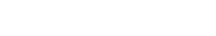withthe velocity
Velocity
In physics, velocity is speed in a given direction. Speed describes only how fast an object is moving, whereas velocity gives both the speed and direction of the object's motion. To have a constant velocity, an object must have a constant speed and motion in a constant direction. Constant ...

,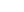the rest mass, and γ the Lorentz factor
Lorentz factor
The Lorentz factor or Lorentz term appears in several equations in special relativity, including time dilation, length contraction, and the relativistic mass formula. Because of its ubiquity, physicists generally represent it with the shorthand symbol γ . It gets its name from its earlier...

.

He included the second term on the right to make sure that for small velocities, the energy would be the same as in classical mechanics: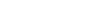Without this second term, there would be an additional contribution in the energy when the particle is not moving.

Einstein found that the total momentum of a moving particle is: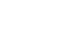and it is this quantity which is conserved in collisions. The ratio of the momentum to the velocity is the relativistic mass, m.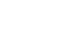And the relativistic mass and the relativistic kinetic energy are related by the formula: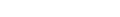Einstein wanted to omit the unnatural second term on the right-hand side, whose only purpose is to make the energy at rest zero, and to declare that the particle has a total energy which obeys:which is a sum of the rest energy m0c2 and the kinetic energy. This total energy is mathematically more elegant, and fits better with the momentum in relativity. But to come to this conclusion, Einstein needed to think carefully about collisions. This expression for the energy implied that matter at rest has a huge amount of energy, and it is not clear whether this energy is physically real, or just a mathematical artifact with no physical meaning.

In a collision process where all the rest-masses are the same at the beginning as at the end, either expression for the energy is conserved. The two expressions only differ by a constant which is the same at the beginning and at the end of the collision. Still, by analyzing the situation where particles are thrown off a heavy central particle, it is easy to see that the inertia of the central particle is reduced by the total energy emitted. This allowed Einstein to conclude that the inertia of a heavy particle is increased or diminished according to the energy it absorbs or emits.

### Relativistic mass

After Einstein first made his proposal, it became clear that the word mass can have two different meanings. The rest mass is what Einstein called m, but others defined the relativistic mass with an explicit index: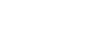This mass is the ratio of momentum to velocity, and it is also the relativistic energy divided by (it is not Lorentz-invariant, in contrast to). The equation holds for moving objects. When the velocity is small, the relativistic mass and the rest mass are almost exactly the same.
• E=mc2 either means E=m0c2 for an object at rest, or E=mrelc2 when the object is moving.

Also Einstein (following Hendrik Lorentz
Hendrik Lorentz
Hendrik Antoon Lorentz was a Dutch physicist who shared the 1902 Nobel Prize in Physics with Pieter Zeeman for the discovery and theoretical explanation of the Zeeman effect...

and Max Abraham
Max Abraham
Max Abraham was a German physicist.Abraham was born in Danzig, Imperial Germany to a family of Jewish merchants. His father was Moritz Abraham and his mother was Selma Moritzsohn. Attending the University of Berlin, he studied under Max Planck. He graduated in 1897...

) used velocity—and direction-dependent mass concepts (longitudinal and transverse mass) in his 1905 electrodynamics paper and in another paper in 1906.
However, in his first paper on E=mc2 (1905), he treated m as what would now be called the rest mass. Some claim that (in later years) he did not like the idea of "relativistic mass."  When modern physicists say "mass", they are usually talking about rest mass, since if they meant "relativistic mass", they would just say "energy".

Considerable debate has ensued over the use of the concept "relativistic mass" and the connection of "mass" in relativity to "mass" in Newtonian dynamics. For example, one view is that only rest mass is a viable concept and is a property of the particle; while relativistic mass is a conglomeration of particle properties and properties of spacetime. A perspective that avoids this debate, due to Kjell Vøyenli, is that the Newtonian concept of mass as a particle property and the relativistic concept of mass have to be viewed as embedded in their own theories and as having no precise connection.

### Low-speed expansion

We can rewrite the expression E = γm0c2 as a Taylor series
Taylor series
In mathematics, a Taylor series is a representation of a function as an infinite sum of terms that are calculated from the values of the function's derivatives at a single point....

: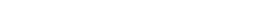For speeds much smaller than the speed of light, higher-order terms in this expression get smaller and smaller because v/c is small. For low speeds we can ignore all but the first two terms: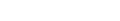The total energy is a sum of the rest energy and the Newtonian
Classical mechanics
In physics, classical mechanics is one of the two major sub-fields of mechanics, which is concerned with the set of physical laws describing the motion of bodies under the action of a system of forces...

kinetic energy
Kinetic energy
The kinetic energy of an object is the energy which it possesses due to its motion.It is defined as the work needed to accelerate a body of a given mass from rest to its stated velocity. Having gained this energy during its acceleration, the body maintains this kinetic energy unless its speed changes...

.

The classical energy equation ignores both the m0c2 part, and the high-speed corrections. This is appropriate, because all the high-order corrections are small. Since only changes in energy affect the behavior of objects, whether we include the m0c2 part makes no difference, since it is constant. For the same reason, it is possible to subtract the rest energy from the total energy in relativity. By considering the emission of energy in different frames, Einstein could show that the rest energy has a real physical meaning.

The higher-order terms are extra correction to Newtonian mechanics which become important at higher speeds. The Newtonian equation is only a low-speed approximation, but an extraordinarily good one. All of the calculations used in putting astronauts on the moon, for example, could have been done using Newton's equations without any of the higher-order corrections.

## History

While Einstein was the first to have correctly deduced the mass–energy equivalence formula, he was not the first to have related energy with mass. But nearly all previous authors thought that the energy which contributes to mass comes only from electromagnetic fields.

### Newton: Matter and light

In 1717 Isaac Newton
Isaac Newton
Sir Isaac Newton PRS was an English physicist, mathematician, astronomer, natural philosopher, alchemist, and theologian, who has been "considered by many to be the greatest and most influential scientist who ever lived."...

speculated that light particles and matter particles were inter-convertible in "Query 30" of the Opticks
Opticks
Opticks is a book written by English physicist Isaac Newton that was released to the public in 1704. It is about optics and the refraction of light, and is considered one of the great works of science in history...

### Electromagnetic rest mass

There were many attempts in the 19th and the beginning of the 20th century—like those of J. J. Thomson
J. J. Thomson
Sir Joseph John "J. J." Thomson, OM, FRS was a British physicist and Nobel laureate. He is credited for the discovery of the electron and of isotopes, and the invention of the mass spectrometer...

(1881), Oliver Heaviside
Oliver Heaviside
Oliver Heaviside was a self-taught English electrical engineer, mathematician, and physicist who adapted complex numbers to the study of electrical circuits, invented mathematical techniques to the solution of differential equations , reformulated Maxwell's field equations in terms of electric and...

(1888), and George Frederick Charles Searle
George Frederick Charles Searle
George Frederick Charles Searle was a British physicist and teacher, and a Fellow of the Royal Society.-Biography:Searle was born in Oakington, Cambridgeshire, England....

(1897)—to understand how the mass of a charged object depends on the electrostatic field. Because the electromagnetic field carries part of the momentum of a moving charge, it was also suspected that the mass of an electron would vary with velocity near the speed of light. Searle calculated that it is impossible for a charged object to supersede the velocity of light because this would require an infinite amount of energy.

Following Thomson and Searle (1896), Wilhelm Wien
Wilhelm Wien
Wilhelm Carl Werner Otto Fritz Franz Wien was a German physicist who, in 1893, used theories about heat and electromagnetism to deduce Wien's displacement law, which calculates the emission of a blackbody at any temperature from the emission at any one reference temperature.He also formulated an...

(1900), Max Abraham
Max Abraham
Max Abraham was a German physicist.Abraham was born in Danzig, Imperial Germany to a family of Jewish merchants. His father was Moritz Abraham and his mother was Selma Moritzsohn. Attending the University of Berlin, he studied under Max Planck. He graduated in 1897...

(1902), and Hendrik Lorentz
Hendrik Lorentz
Hendrik Antoon Lorentz was a Dutch physicist who shared the 1902 Nobel Prize in Physics with Pieter Zeeman for the discovery and theoretical explanation of the Zeeman effect...

(1904) argued that this relation applies to the complete mass of bodies, because all inertial mass is electromagnetic in origin. The formula of the mass–energy-relation given by them was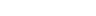. Wien went on by stating, that if it is assumed that gravitation is an electromagnetic effect too, then there has to be a strict proportionality between (electromagnetic) inertial mass and (electromagnetic) gravitational mass. This interpretation is in the now discredited electromagnetic worldview, and the formulas that they discovered always included a factor of 4/3 in the proportionality. For example, the formulas given by Lorentz in 1904 for the pre-relativistic longitudinal and transverse masses were (in modern notation):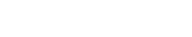,

where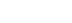In July 1905 (published 1906), nearly at the same time when Einstein found the simple relation from relativity, Poincaré was able to explain the reason that the electromagnetic mass calculations always had a factor of 4/3. In order for a particle consisting of positive or negative charge to be stable, there must be some sort of attractive force of non-electrical nature which keeps it together. If the mass–energy of this force field is included in a way which is consistent with relativity theory, the attractive contribution adds an amountto the energy of the bodies, and this explains the discrepancy between the pure electromagnetic theory and relativity.

### Inertia of energy and radiation

James Clerk Maxwell
James Clerk Maxwell
James Clerk Maxwell of Glenlair was a Scottish physicist and mathematician. His most prominent achievement was formulating classical electromagnetic theory. This united all previously unrelated observations, experiments and equations of electricity, magnetism and optics into a consistent theory...

Adolfo Bartoli was an Italian physicist, who is best known for introducing the concept of radiation pressure from thermodynamical considerations....

(1876) found out that the existence of tensions in the ether like the radiation pressure
Radiation pressure is the pressure exerted upon any surface exposed to electromagnetic radiation. If absorbed, the pressure is the power flux density divided by the speed of light...

follows from the electromagnetic theory. However, Lorentz (1895) recognized that this led to a conflict between the action/reaction principle
Newton's laws of motion
Newton's laws of motion are three physical laws that form the basis for classical mechanics. They describe the relationship between the forces acting on a body and its motion due to those forces...

and Lorentz's ether theory
Lorentz ether theory
What is now often called Lorentz Ether theory has its roots in Hendrik Lorentz's "Theory of electrons", which was the final point in the development of the classical aether theories at the end of the 19th and at the beginning of the 20th century....

.

#### Poincaré

In 1900 Henri Poincaré
Henri Poincaré
Jules Henri Poincaré was a French mathematician, theoretical physicist, engineer, and a philosopher of science...

studied this conflict and tried to determine whether the center of gravity
Center of gravity
In physics, a center of gravity of a material body is a point that may be used for a summary description of gravitational interactions. In a uniform gravitational field, the center of mass serves as the center of gravity...

still moves with a uniform velocity when electromagnetic fields are included. He noticed that the action/reaction principle does not hold for matter alone, but that the electromagnetic field has its own momentum. The electromagnetic field energy behaves like a fictitious fluid
Fluid
In physics, a fluid is a substance that continually deforms under an applied shear stress. Fluids are a subset of the phases of matter and include liquids, gases, plasmas and, to some extent, plastic solids....

("fluide fictif") with a mass density of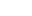(in other words m = E/c2). If the center of mass frame is defined by both the mass of matter and the mass of the fictitious fluid, and if the fictitious fluid is indestructible—it is neither created or destroyed—then the motion of the center of mass frame remains uniform. But electromagnetic energy can be converted into other forms of energy. So Poincaré assumed that there exists a non-electric energy fluid at each point of space, into which electromagnetic energy can be transformed and which also carries a mass proportional to the energy. In this way, the motion of the center of mass remains uniform. Poincaré said that one should not be too surprised by these assumptions, since they are only mathematical fictions.

But Poincaré's resolution led to a paradox when changing frames: if a Hertzian oscillator radiates in a certain direction, it will suffer a recoil
Recoil
Recoil is the backward momentum of a gun when it is discharged. In technical terms, the recoil caused by the gun exactly balances the forward momentum of the projectile and exhaust gasses, according to Newton's third law...

from the inertia of the fictitious fluid. In the framework of Lorentz ether theory
Lorentz ether theory
What is now often called Lorentz Ether theory has its roots in Hendrik Lorentz's "Theory of electrons", which was the final point in the development of the classical aether theories at the end of the 19th and at the beginning of the 20th century....

Poincaré performed a Lorentz boost to the frame of the moving source. He noted that energy conservation holds in both frames, but that the law of conservation of momentum is violated. This would allow a perpetuum mobile
Perpetual motion
Perpetual motion describes hypothetical machines that operate or produce useful work indefinitely and, more generally, hypothetical machines that produce more work or energy than they consume, whether they might operate indefinitely or not....

, a notion which he abhorred. The laws of nature would have to be different in the frames of reference, and the relativity principle would not hold. Poincaré's paradox was resolved by Einstein's insight that a body losing energy as radiation or heat was losing a mass of the amount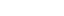. The Hertzian oscillator loses mass in the emission process, and momentum is conserved in any frame. Einstein noted in 1906 that Poincaré's solution to the center of mass problem and his own were mathematically equivalent (see below).

Poincaré came back to this topic in "Science and Hypothesis" (1902) and "The Value of Science
The Value of Science
The Value of Science is a book by the French mathematician, physicist, and philosopher Henri Poincaré. It was published in 1905. The book deals with questions in the philosophy of science and adds detail to the topics addressed by Poincaré's previous book, Science and Hypothesis .-Intuition and...

" (1905). This time he rejected the possibility that energy carries mass: "... [the recoil] is contrary to the principle of Newton since our projectile here has no mass, it is not matter, it is energy". He also discussed two other unexplained effects: (1) non-conservation of mass implied by Lorentz's variable mass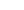, Abraham's theory of variable mass and Kaufmann
Walter Kaufmann (physicist)
Walter Kaufmann was a German physicist. He is most well known for his first experimental proof of the velocity dependence of mass, which was an important contribution to the development of modern physics, including special relativity.-Life:In 1890/91 he studied mechanical engineering at the...

's experiments on the mass of fast moving electrons and (2) the non-conservation of energy in the radium experiments of Madame Curie.

#### Abraham and Hasenöhrl

Following Poincaré, Max Abraham
Max Abraham
Max Abraham was a German physicist.Abraham was born in Danzig, Imperial Germany to a family of Jewish merchants. His father was Moritz Abraham and his mother was Selma Moritzsohn. Attending the University of Berlin, he studied under Max Planck. He graduated in 1897...

in 1902 introduced the term "electromagnetic momentum" to maintain the action/reaction principle. Poincaré's result was verified by him, whereby the field density of momentum per cm3 isand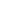per cm2.

In 1904, Friedrich Hasenöhrl
Friedrich Hasenöhrl
Friedrich Hasenöhrl , was an Austro-Hungarian physicist.-Life:Friedrich Hasenöhrl was born in Vienna, Austria in 1874. His father was a lawyer and his mother belonged to a prominent aristocratic family...

specifically associated inertia with radiation in a paper, which was according to his own words very similar to some papers of Abraham. Hasenöhrl suggested that part of the mass of a body (which he called apparent mass) can be thought of as radiation bouncing around a cavity. The apparent mass of radiation depends on the temperature (because every heated body emits radiation) and is proportional to its energy, and he first concluded that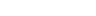. However, in 1905 Hasenöhrl published a summary of a letter, which was written by Abraham to him. Abraham concluded that Hasenöhrl's formula of the apparent mass of radiation is not correct, and on the basis of his definition of electromagnetic momentum and longitudinal electromagnetic mass Abraham changed it to, the same value for the electromagnetic mass for a body at rest. Hasenöhrl re-calculated his own derivation and verified Abraham's result. He also noticed the similarity between the apparent mass and the electromagnetic mass. However, Hasenöhrl stated that this energy–apparent-mass relation only holds as long a body radiates, i.e. if the temperature of a body is greater than 0 K
Kelvin
The kelvin is a unit of measurement for temperature. It is one of the seven base units in the International System of Units and is assigned the unit symbol K. The Kelvin scale is an absolute, thermodynamic temperature scale using as its null point absolute zero, the temperature at which all...

.

However, Hasenöhrl did not include the pressure of the radiation on the cavity shell. If he had included the shell pressure and inertia as it would be included in the theory of relativity, the factor would have been equal to 1 or. This calculation assumes that the shell properties are consistent with relativity, otherwise the mechanical properties of the shell including the mass and tension would not have the same transformation laws as those for the radiation. Nobel Prize
Nobel Prize
The Nobel Prizes are annual international awards bestowed by Scandinavian committees in recognition of cultural and scientific advances. The will of the Swedish chemist Alfred Nobel, the inventor of dynamite, established the prizes in 1895...

-winner and Hitler
Adolf Hitler was an Austrian-born German politician and the leader of the National Socialist German Workers Party , commonly referred to as the Nazi Party). He was Chancellor of Germany from 1933 to 1945, and head of state from 1934 to 1945...

Philipp Lenard
Philipp Eduard Anton von Lenard , known in Hungarian as Lénárd Fülöp Eduárd Antal, was a Hungarian - German physicist and the winner of the Nobel Prize for Physics in 1905 for his research on cathode rays and the discovery of many of their properties...

claimed that the mass–energy equivalence formula needed to be credited to Hasenöhrl to make it an Aryan creation.

### Einstein: mass–energy equivalence

Albert Einstein
Albert Einstein
Albert Einstein was a German-born theoretical physicist who developed the theory of general relativity, effecting a revolution in physics. For this achievement, Einstein is often regarded as the father of modern physics and one of the most prolific intellects in human history...

did not formulate exactly the formula in his 1905 Annus Mirabilis paper
Annus Mirabilis Papers
The Annus Mirabilis papers are the papers of Albert Einstein published in the Annalen der Physik scientific journal in 1905. These four articles contributed substantially to the foundation of modern physics and changed views on space, time, and matter...

"Does the Inertia of a Body Depend Upon Its Energy Content?"; rather, the paper states that if a body gives off the energy L in the form of radiation, its mass diminishes by L/c2. (Here, "radiation" means electromagnetic radiation
Electromagnetic radiation is a form of energy that exhibits wave-like behavior as it travels through space...

, or light, and mass means the ordinary Newtonian mass of a slow-moving object.) This formulation relates only a change Δm in mass to a change L in energy without requiring the absolute relationship.

Objects with zero mass presumably have zero energy, so the extension that all mass is proportional to energy is obvious from this result. In 1905, even the hypothesis that changes in energy are accompanied by changes in mass was untested. Not until the discovery of the first type of antimatter (the positron
Positron
The positron or antielectron is the antiparticle or the antimatter counterpart of the electron. The positron has an electric charge of +1e, a spin of ½, and has the same mass as an electron...

in 1932) was it found that all of the mass of pairs of resting particles could be converted to radiation.

#### First correct derivation (1905)

The correctness of Einstein's 1905 derivation of E=mc2 was criticized by Max Planck
Max Planck
Max Karl Ernst Ludwig Planck, ForMemRS, was a German physicist who actualized the quantum physics, initiating a revolution in natural science and philosophy. He is regarded as the founder of the quantum theory, for which he received the Nobel Prize in Physics in 1918.-Life and career:Planck came...

(1907), and also by Herbert Ives (1952), and also in a recent book (2008) by Hans Ohanian.

Einstein considered a body at rest with mass M. If the body is examined in a frame moving with nonrelativistic velocity v, it is no longer at rest and in the moving frame it has momentum P = Mv.

Einstein supposed the body emits two pulses of light to the left and to the right, each carrying an equal amount of energy E/2. In its rest frame, the object remains at rest after the emission since the two beams are equal in strength and carry opposite momentum.

But if the same process is considered in a frame moving with velocity v to the left, the pulse moving to the left will be redshift
Redshift
In physics , redshift happens when light seen coming from an object is proportionally increased in wavelength, or shifted to the red end of the spectrum...

ed while the pulse moving to the right will be blue shift
Blue shift
A blueshift is any decrease in wavelength ; the opposite effect is referred to as redshift. In visible light, this shifts the colour from the red end of the spectrum to the blue end...

ed. The blue light carries more momentum than the red light, so that the momentum of the light in the moving frame is not balanced: the light is carrying some net momentum to the right.

The object has not changed its velocity before or after the emission. Yet in this frame it has lost some right-momentum to the light. The only way it could have lost momentum is by losing mass. This also solves Poincaré's radiation paradox, discussed above.

The velocity is small, so the right-moving light is blueshifted by an amount equal to the nonrelativistic Doppler shift factor . The momentum of the light is its energy divided by c, and it is increased by a factor of v/c. So the right-moving light is carrying an extra momentum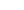given by: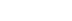The left-moving light carries a little less momentum, by the same amount. So the total right-momentum in the light is twice. This is the right-momentum that the object lost.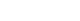The momentum of the object in the moving frame after the emission is reduced by this amount: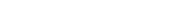So the change in the object's mass is equal to the total energy lost divided by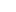. Since any emission of energy can be carried out by a two step process, where first the energy is emitted as light and then the light is converted to some other form of energy, any emission of energy is accompanied by a loss of mass. Similarly, by considering absorption, a gain in energy is accompanied by a gain in mass. Einstein concludes that the mass of a body is a measure of its energy content.

#### Relativistic center-of-mass theorem – 1906

Like Poincaré, Einstein concluded in 1906 that the inertia of electromagnetic energy is a necessary condition for the center-of-mass theorem to hold. On this occasion, Einstein referred to Poincaré's 1900 paper and wrote:
In Einstein's more physical, as opposed to formal or mathematical, point of view, there was no need for fictitious masses. He could avoid the perpetuum mobile
Perpetual motion
Perpetual motion describes hypothetical machines that operate or produce useful work indefinitely and, more generally, hypothetical machines that produce more work or energy than they consume, whether they might operate indefinitely or not....

problem, because on the basis of the mass–energy equivalence he could show that the transport of inertia which accompanies the emission and absorption of radiation solves the problem. Poincaré's rejection of the principle of action–reaction can be avoided through Einstein's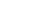, because mass conservation appears as a special case of the energy conservation law.

### Others

During the nineteenth century there were several speculative attempts to show that mass and energy were proportional in various ether theories. In 1873 Nikolay Umov
Nikolay Umov
Nikolay Alekseevich Umov was a Russian physicist and mathematician known for discovering the concept of Umov-Poynting vector and Umov effect.-Biography:...

pointed out a relation between mass and energy for ether in the form of Е = kmc2, where 0.5 ≤ k ≤ 1. The writings of Samuel Tolver Preston
Samuel Tolver Preston
Samuel Tolver Preston was an English Engineer and physicist.His parents were Daniel Bloom Preston and Mary Susannah Tolver. Preston was educated as a Telegraph-engineer. He went to Munich where he attained his Ph.D in 1894 with Ludwig Boltzmann...

, and a 1903 paper by Olinto De Pretto
Olinto De Pretto
Olinto De Pretto was an Italian industrialist, physicist and geologist from Schio, Vicenza. He is credited with being the first person to derive the precise formula E=mc2 and is also the first to conclude that radioactive decay of uranium and thorium was an example of energy transformation from...

, presented a mass–energy relation. De Pretto's paper received recent press coverage when Umberto Bartocci discovered that there were only three degrees of separation
Six degrees of separation
Six degrees of separation refers to the idea that everyone is on average approximately six steps away, by way of introduction, from any other person on Earth, so that a chain of, "a friend of a friend" statements can be made, on average, to connect any two people in six steps or fewer...

linking De Pretto to Einstein, leading Bartocci to conclude that Einstein was probably aware of De Pretto's work.

Preston and De Pretto, following Le Sage
Le Sage's theory of gravitation
Le Sage's theory of gravitation is a kinetic theory of gravity originally proposed by Nicolas Fatio de Duillier in 1690 and later by Georges-Louis Le Sage in 1748. The theory proposed a mechanical explanation for Newton's gravitational force in terms of streams of tiny unseen particles impacting...

, imagined that the universe was filled with an ether of tiny particles which are always moving at speed c. Each of these particles have a kinetic energy of mc2 up to a small numerical factor. The nonrelativistic kinetic energy formula did not always include the traditional factor of 1/2, since Leibniz
Gottfried Leibniz
Gottfried Wilhelm Leibniz was a German philosopher and mathematician. He wrote in different languages, primarily in Latin , French and German ....

introduced kinetic energy without it, and the 1/2 is largely conventional in prerelativistic physics. By assuming that every particle has a mass which is the sum of the masses of the ether particles, the authors would conclude that all matter contains an amount of kinetic energy either given by E = mc2 or 2E = mc2 depending on the convention. A particle ether was usually considered unacceptably speculative science at the time, and since these authors did not formulate relativity, their reasoning is completely different from that of Einstein, who used relativity to change frames.

Independently, Gustave Le Bon
Gustave Le Bon
Gustave Le Bon was a French social psychologist, sociologist, and amateur physicist...

in 1905 speculated that atoms could release large amounts of latent energy, reasoning from an all-encompassing qualitative philosophy of physics.

It was quickly noted after the discovery of radioactivity in 1897, that the total energy due to radioactive processes is about one million times greater than that involved in any known molecular change. However, it raised the question where this energy is coming from. After eliminating the idea of absorption and emission of some sort of Lesagian ether particles
Le Sage's theory of gravitation
Le Sage's theory of gravitation is a kinetic theory of gravity originally proposed by Nicolas Fatio de Duillier in 1690 and later by Georges-Louis Le Sage in 1748. The theory proposed a mechanical explanation for Newton's gravitational force in terms of streams of tiny unseen particles impacting...

, the existence of a huge amount of latent energy, stored within matter, was proposed by Ernest Rutherford
Ernest Rutherford
Ernest Rutherford, 1st Baron Rutherford of Nelson OM, FRS was a New Zealand-born British chemist and physicist who became known as the father of nuclear physics...

and Frederick Soddy
Frederick Soddy
Frederick Soddy was an English radiochemist who explained, with Ernest Rutherford, that radioactivity is due to the transmutation of elements, now known to involve nuclear reactions. He also proved the existence of isotopes of certain radioactive elements...

in 1903. Rutherford also suggested that this internal energy is stored within normal matter as well. He went on to speculate in 1904:
Einstein's equation is in no way an explanation of the large energies released in radioactive decay (this comes from the powerful nuclear force
Nuclear force
The nuclear force is the force between two or more nucleons. It is responsible for binding of protons and neutrons into atomic nuclei. The energy released causes the masses of nuclei to be less than the total mass of the protons and neutrons which form them...

s involved; forces that were still unknown in 1905). In any case, the enormous energy released from radioactive decay (which had been measured by Rutherford) was much more easily measured than the (still small) change in the gross mass of materials, as a result. Einstein's equation, by theory, can give these energies by measuring mass differences before and after reactions, but in practice, these mass differences in 1905 were still too small to be measured in bulk. Prior to this, the ease of measuring radioactive decay energies with a calorimeter was thought possibly likely to allow measurement of changes in mass difference, as a check on Einstein's equation itself. Einstein mentions in his 1905 paper that mass–energy equivalence might perhaps be tested with radioactive decay, which releases enough energy (the quantitative amount known roughly by 1905) to possibly be "weighed," when missing from the system (having been given off as heat). However, radioactivity seemed to proceed at its own unalterable (and quite slow, for radioactives known then) pace, and even when simple nuclear reactions became possible using proton bombardment, the idea that these great amounts of usable energy could be liberated at will with any practicality, proved difficult to substantiate. It had been used as the basis of much speculation, causing Rutherford himself to later reject his ideas of 1904; he was reported in 1933 to have said that: "Anyone who expects a source of power from the transformation of the atom is talking moonshine
Moonshine
Moonshine is an illegally produced distilled beverage...

."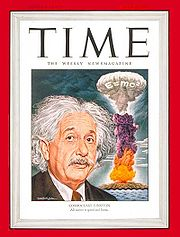This situation changed dramatically in 1932 with the discovery of the neutron and its mass, allowing mass differences for single nuclide
Nuclide
A nuclide is an atomic species characterized by the specific constitution of its nucleus, i.e., by its number of protons Z, its number of neutrons N, and its nuclear energy state....

s and their reactions to be calculated directly, and compared with the sum of masses for the particles that made up their composition. In 1933, the energy released from the reaction of lithium-7 plus protons giving rise to 2 alpha particles (as noted above by Rutherford), allowed Einstein's equation to be tested to an error of ± 0.5%. However, scientists still did not see such reactions as a source of power.

After the very public demonstration of huge energies released from nuclear fission
Nuclear fission
In nuclear physics and nuclear chemistry, nuclear fission is a nuclear reaction in which the nucleus of an atom splits into smaller parts , often producing free neutrons and photons , and releasing a tremendous amount of energy...

after the atomic bombings of Hiroshima and Nagasaki
Atomic bombings of Hiroshima and Nagasaki
During the final stages of World War II in 1945, the United States conducted two atomic bombings against the cities of Hiroshima and Nagasaki in Japan, the first on August 6, 1945, and the second on August 9, 1945. These two events are the only use of nuclear weapons in war to date.For six months...

in 1945, the equation E = mc2 became directly linked in the public eye with the power and peril of nuclear weapon
Nuclear weapon
A nuclear weapon is an explosive device that derives its destructive force from nuclear reactions, either fission or a combination of fission and fusion. Both reactions release vast quantities of energy from relatively small amounts of matter. The first fission bomb test released the same amount...

s. The equation was featured as early as page 2 of the Smyth Report
Smyth Report
The Smyth Report was the common name given to an administrative history written by physicist Henry DeWolf Smyth about the Allied World War II effort to develop the atomic bomb, the Manhattan Project...

, the official 1945 release by the US government on the development of the atomic bomb, and by 1946 the equation was linked closely enough with Einstein's work that the cover of Time
Time (magazine)
Time is an American news magazine. A European edition is published from London. Time Europe covers the Middle East, Africa and, since 2003, Latin America. An Asian edition is based in Hong Kong...

magazine prominently featured a picture of Einstein next to an image of a mushroom cloud
Mushroom cloud
A mushroom cloud is a distinctive pyrocumulus mushroom-shaped cloud of condensed water vapor or debris resulting from a very large explosion. They are most commonly associated with nuclear explosions, but any sufficiently large blast will produce the same sort of effect. They can be caused by...

emblazoned with the equation. Einstein himself had only a minor role in the Manhattan Project
Manhattan Project
The Manhattan Project was a research and development program, led by the United States with participation from the United Kingdom and Canada, that produced the first atomic bomb during World War II. From 1942 to 1946, the project was under the direction of Major General Leslie Groves of the US Army...

: he had cosigned a letter to the U.S. President in 1939 urging funding for research into atomic energy, warning that an atomic bomb was theoretically possible. The letter persuaded Roosevelt to devote a significant portion of the wartime budget to atomic research. Without a security clearance, Einstein's only scientific contribution was an analysis of an isotope separation
Isotope separation
Isotope separation is the process of concentrating specific isotopes of a chemical element by removing other isotopes, for example separating natural uranium into enriched uranium and depleted uranium. This is a crucial process in the manufacture of uranium fuel for nuclear power stations, and is...

method in theoretical terms. It was inconsequential, on account of Einstein not being given sufficient information (for security reasons) to fully work on the problem.

While E = mc2 is useful for understanding the amount of energy potentially released in a fission reaction, it was not strictly necessary to develop the weapon, once the fission process was known, and its energy measured at 200 MeV (which was directly possible, using a quantitative Geiger counter, at that time). As the physicist and Manhattan Project participant Robert Serber
Robert Serber
Robert Serber was an American physicist who participated in the Manhattan Project. He was born in Philadelphia, Pennsylvania; he was the eldest son of David Serber and Rose Frankel. He married Charlotte Leof in 1933. Rose Serber died in 1922; David married Charlotte's cousin Frances Leof in...

put it: "Somehow the popular notion took hold long ago that Einstein's theory of relativity, in particular his famous equation E = mc2, plays some essential role in the theory of fission. Albert Einstein had a part in alerting the United States government to the possibility of building an atomic bomb, but his theory of relativity is not required in discussing fission. The theory of fission is what physicists call a non-relativistic theory, meaning that relativistic effects are too small to affect the dynamics of the fission process significantly." However the association between E = mc2 and nuclear energy has since stuck, and because of this association, and its simple expression of the ideas of Albert Einstein himself, it has become "the world's most famous equation".

While Serber's view of the strict lack of need to use mass–energy equivalence in designing the atomic bomb is correct, it does not take into account the pivotal role which this relationship played in making the fundamental leap to the initial hypothesis that large atoms were energetically allowed to split into approximately equal parts (before this energy was in fact measured). In late 1938, while on the winter walk on which they solved the meaning of Hahn's experimental results and introduced the idea that would be called atomic fission, Lise Meitner
Lise Meitner
Lise Meitner FRS was an Austrian-born, later Swedish, physicist who worked on radioactivity and nuclear physics. Meitner was part of the team that discovered nuclear fission, an achievement for which her colleague Otto Hahn was awarded the Nobel Prize...

and Otto Robert Frisch
Otto Robert Frisch
Otto Robert Frisch , Austrian-British physicist. With his collaborator Rudolf Peierls he designed the first theoretical mechanism for the detonation of an atomic bomb in 1940.- Overview :...

made direct use of Einstein's equation to help them understand the quantitative energetics of the reaction which overcame the "surface tension-like" forces holding the nucleus together, and allowed the fission fragments to separate to a configuration from which their charges could force them into an energetic "fission". To do this, they made use of "packing fraction", or nuclear binding energy
Binding energy
Binding energy is the mechanical energy required to disassemble a whole into separate parts. A bound system typically has a lower potential energy than its constituent parts; this is what keeps the system together—often this means that energy is released upon the creation of a bound state...

values for elements, which Meitner had memorized. These, together with use of E = mc2 allowed them to realize on the spot that the basic fission process was energetically possible:

...We walked up and down in the snow, I on skis and she on foot. ...and gradually the idea took shape... explained by Bohr's idea that the nucleus is like a liquid drop; such a drop might elongate and divide itself... We knew there were strong forces that would resist, ..just as surface tension. But nuclei differed from ordinary drops. At this point we both sat down on a tree trunk and started to calculate on scraps of paper. ...the Uranium nucleus might indeed be a ginger kid, ready to divide itself... But, ...when the two drops separated they would be driven apart by electrical repulsion, about 200 MeV in all. Fortunately Lise Meitner remembered how to compute the masses of nuclei... and worked out that the two nuclei formed... would be lighter by about one-fifth the mass of a proton. Now whenever mass disappears energy is created, according to Einstein's formula E = mc2, and... the mass was just equivalent to 200 MeV; it all fitted!

• Binding energy
Binding energy
Binding energy is the mechanical energy required to disassemble a whole into separate parts. A bound system typically has a lower potential energy than its constituent parts; this is what keeps the system together—often this means that energy is released upon the creation of a bound state...

(mass defect)
• Energy density
Energy density
Energy density is a term used for the amount of energy stored in a given system or region of space per unit volume. Often only the useful or extractable energy is quantified, which is to say that chemically inaccessible energy such as rest mass energy is ignored...

• Energy–momentum relation
• Inertia
Inertia
Inertia is the resistance of any physical object to a change in its state of motion or rest, or the tendency of an object to resist any change in its motion. It is proportional to an object's mass. The principle of inertia is one of the fundamental principles of classical physics which are used to...

• Mass in special relativity
Mass in special relativity
Mass in special relativity incorporates the general understandings from the concept of mass-energy equivalence. Added to this concept is an additional complication resulting from the fact that "mass" is defined in two different ways in special relativity: one way defines mass as an invariant...

• Mass, momentum, and energy
• Index of energy articles
Index of energy articles
This is a index of energy articles.-A:Alternative energy- Alternative energy indexes- American Museum of Science and Energy - Anisotropy energy-E:Ecological energetics- Electric potential energy- Energy- Energy accounting...

• list of wave topics
• outline of energy